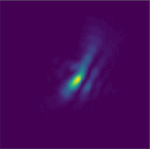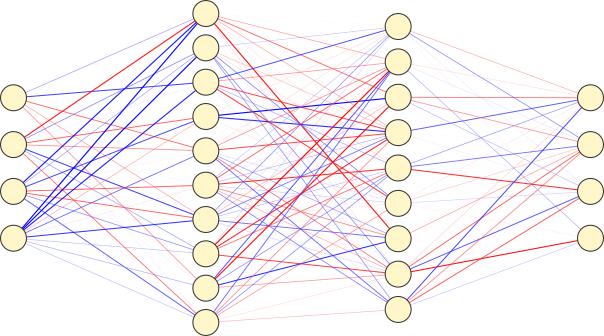Created by sebastien.popoff on 07/08/2019

# Setting up a DMD/SLM: Aberration effectsDigital Micromirror Devices (DMDs) are amplitude only (binary) modulators, however, pretty much like liquid crystal modulators, they introduce some phase distortion. Practically, it means that if one illuminates the modulator with a plane wave, even when all the pixels are set to the same value, the wavefront shows phase distortions after reflection. That can be detrimental, especially when working in a plane conjugated with the Fourier plane of the DMD surface. Fortunately, using the Lee hologram method or the superpixel method, one can achieve phase modulation. I present here how to use Lee holograms to characterize and compensate for aberrations when using a DMD. This approach can also be applied for compensating for aberration effects in other types of Spatial Light Modulators, such as liquid crystal ones.

Created by sebastien.popoff on 26/05/2019

## Compare Different Methods of Modes Estimation of Bent Multimode Fibers with pyMMFIn a previous tutorial, I explained how to calculate the modes of a bent multimode fibers. I introduced two methods, following the approach published in [M. Plöschner, T. Tyc, and T. Čižmár, Nat. Photon. (2015)]. In this short tutorial, I show how to use pyMMF to simulate bent fibers and compare the two different methods. A Jupyter notebook can be found on my Github account: compare_bending_methods.ipynb.

Created by sebastien.popoff on 22/05/2019

## Semidefinite Programming for Intensity Only Estimation of the Transmission Matrix

The possibility of measuring the transmission matrix using intensity only measurements is a much sought-after feature as it allows us not to rely on interferometry. Interferometry usually requires laboratory-grade stability difficult to obtain for real-world applications. Typically, we want to be able to retrieve the transmission matrix from a set of pairs composed of input masks and output intensity patterns. However, this problem, which corresponds to a phase-retrieval problem, is not convex, hence difficult to solve using standard techniques. The idea proposed in [I. Waldspurger et al., Math. Program (2015)] is to relax some constraints to approximate the problem to a convex one that can be solved using the semidefinite programming approach. I briefly detail the approach and provide an example of the procedure to reconstruct the transmission matrix using Python. A Jupyter notebook can be found on my Github account: semidefiniteTM_example.ipynb.

Created by sebastien.popoff on 19/05/2019

## An implementation in PyTorchArtificial neural networks are mainly used for treating data encoded in real values, such as digitized images or sounds. In such systems, using complex-valued tensors would be quite useless. This is however different for physic related topics. When dealing with wave propagation in particular, using complex values is interesting since the physics typically has linear, hence more simple, behavior when considering complex fields. This is sometimes true even when the inputs and the outputs of the system are real values. For instance, consider a complex media that you excite using an amplitude modulator, such as a DMD (Digital Micromirror Device) and you measure the output intensity. You manipulate only real values, but if you want to characterize the system, you have to keep in mind that the phase is a hidden variable as the effect of propagation is represented by the multiplication by a complex matrix on the optical field.